# On the Concept of a Notational Variant

• 1057 Accesses

Part of the Lecture Notes in Computer Science book series (LNTCS,volume 10455)

## Abstract

In the study of modal and nonclassical logics, translations have frequently been employed as a way of measuring the inferential capabilities of a logic. It is sometimes claimed that two logics are “notational variants” if they are translationally equivalent. However, we will show that this cannot be quite right, since first-order logic and propositional logic are translationally equivalent. Others have claimed that for two logics to be notational variants, they must at least be compositionally intertranslatable. The definition of compositionality these accounts use, however, is too strong, as the standard translation from modal logic to first-order logic is not compositional in this sense. In light of this, we will explore a weaker version of this notion that we will call schematicity and show that there is no schematic translation either from first-order logic to propositional logic or from intuitionistic logic to classical logic.

### Keywords

• Translation
• Notational variant
• Lindenbaum-Tarski algebras
• Compositionality
• Schematicity

This is a preview of subscription content, access via your institution.

Chapter
USD   29.95
Price excludes VAT (USA)
• Available as PDF
• Own it forever
eBook
USD   39.99
Price excludes VAT (USA)
• Available as EPUB and PDF
• Own it forever
Softcover Book
USD   54.99
Price excludes VAT (USA)
• Compact, lightweight edition
• Dispatched in 3 to 5 business days
• Free shipping worldwide - see info

Tax calculation will be finalised at checkout

Purchases are for personal use only

1. 1.

For claims like this, see [16, p. 67], [5, p. 391], [12, p. 269], [3, p. 108], [13, p. 139], [11, p. 7] and [6, p. 134].

2. 2.

E.g., [13, p. 139], [11, p. 7] and [6, p. 134].

3. 3.

E.g., [16, p. 67], [5, p. 391], [12, p. 269] and [3, p. 108].

4. 4.

This thereby answers a question posed by Epstein [5, p. 388] in the affirmative. It is also straightforward to generate artificial counterexamples using any two partial orders such that neither is order-embeddable in the other.

5. 5.

See, e.g., [12, p. 269], [3, p. 108], [13, p. 139] and [6, p. 134].

6. 6.

[5, p. 391] uses the term “grammatical” instead of “compositional”. [9, p. 100], [12, p. 269] and [3, p. 108] build compositionality into the definition of a translation from the start.

7. 7.

This follows from Theorems 23 and 24 below. There are also more direct proofs of these claims. For instance, suppose there were a compositional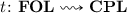. Then where $$\varTheta$$ is the $${\mathbf{{CPL}}}$$-schema such that, we have. Hence,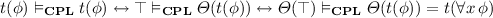for any $$\phi \in \mathcal {L}_\text {pred}$$. But then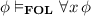for any $$\phi \in \mathcal {L}_\text {pred}$$,.

8. 8.

See, e.g., [1, p. 1] and [2, p. 70].

9. 9.

Mossakowski et al. [11, p. 4] make this observation as well, though they do not offer any alternative notion in its place.

10. 10.

The theorem cannot be extended to all normal modal logics, since there is a compositional translation from $$\mathbf S5$$ to $${\mathbf{{FOL}}}$$ (setting). It is unknown whether the result extends to other logics like $$\mathbf S4$$ that validate.

11. 11.

The definition is inspired by the definition of “recursive” translations from [6, p. 16], who attributes the definition to Steven Kuhn.

12. 12.

We could also require schematic translations to translate atomic formulas schematically. Such a constraint seems well-motivated, but it was not included in this definition for purposes of generality, as it was not necessary in the results to follow.

## References

1. Andréka, H., István, N., van Benthem, J.F.A.K.: Modal languages and bounded fragments of predicate logic. J. Philos. Logic 27(3), 217–274 (1998)

2. Blackburn, P., de Rijke, M., Venema, Y.: Modal Logic. Cambridge University Press, Cambridge (2001)

3. Caleiro, C., Gonçalves, R.: Equipollent logical systems. In: Beziau, J.Y. (ed.) Logica Universalis, pp. 97–109. Springer, Heidelberg (2007). doi:10.1007/978-3-7643-8354-1_6

4. Carnielli, W.A., Coniglio, M.E., D’Ottaviano, I.M.L.: New dimensions on translations between logics. Logica Universalis 3, 1–18 (2009)

5. Epstein, R.L.: The semantic foundations of logic. In: Epstein, R.L. (ed.) The Semantic Foundations of Logic Volume 1: Propositional Logics. Springer, Dordrecht (1990). doi:10.1007/978-94-009-0525-2_11

6. French, R.: Translational embeddings in modal logic. Ph.D. thesis (2010)

7. Gödel, K.: Zur Intuitionistischen Arithmetik und Zahlentheorie. Ergebnisse eines mathematischen Kolloquiums 4, 34–38 (1933). Reprinted in Gödel 1986, pp. 286–295

8. Gödel, K.: Collected Works. Oxford University Press, Oxford (1986)

9. Kracht, M., Wolter, F.: Normal monomodal logics can simulate all others. J. Symb. Logic 64(01), 99–138 (1999)

10. Jeřábek, E.: The ubiquity of conservative translations. Rev. Symb. Logic 5, 666–678 (2012)

11. Mossakowski, T., Diaconescu, R., Tarlecki, A.: What is a logic translation? Logica Universalis 3, 95–124 (2009)

12. Pelletier, F.J., Urquhart, A.: Synonymous logics. J. Philos. Logic 32, 259–285 (2003)

13. Straßburger, L.: What is a logic, and what is a proof? In: Beziau, J.Y. (ed.) Logica Universalis, pp. 135–152. Springer, Basel (2007)

14. Thomason, S.K.: Reduction of tense logic to modal logic. I. J. Symb. Logic 39(3), 549–551 (1974)

15. Thomason, S.K.: Reduction of tense logic to modal logic II. Theoria 41(3), 154–169 (1975)

16. Wójcicki, R.: Theory of Logical Calculi: Basic Theory of Consequence Operators. Springer, Dordrecht (1988). doi:10.1007/978-94-015-6942-2

## Author information

Authors

### Corresponding author

Correspondence to Alexander W. Kocurek .

## A Proof of Theorem 21

### A Proof of Theorem 21

Let $$\varTheta (\xi )$$ be a first-order schema such that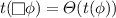. Without loss of generality, we may assume $$\varTheta (\xi )$$ is in (roughly) prenex normal form, i.e., that: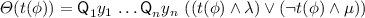where $$\lambda$$ and $$\mu$$ are boolean combinations of atomic $${\mathbf{{FOL}}}$$-formulas and each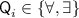. Observe that:So.

First, we show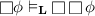. Using the fact that:since $$y_1,\dots ,y_n$$ are already bound in. So:Hence,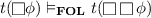, and thus,.

Next, we show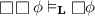. Observe that: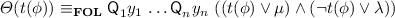So:Thus, in particular,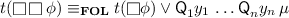. Now, note that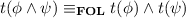. Hence, unpacking:Since, and since(given the last equivalence above), that means thatfor any $$\phi$$ and $$\psi$$. In particular,. Hence,. Thus, we have that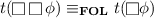.    $$\square$$

## Rights and permissions

Reprints and Permissions

### Cite this paper

Kocurek, A.W. (2017). On the Concept of a Notational Variant. In: Baltag, A., Seligman, J., Yamada, T. (eds) Logic, Rationality, and Interaction. LORI 2017. Lecture Notes in Computer Science(), vol 10455. Springer, Berlin, Heidelberg. https://doi.org/10.1007/978-3-662-55665-8_20

• DOI: https://doi.org/10.1007/978-3-662-55665-8_20

• Published:

• Publisher Name: Springer, Berlin, Heidelberg

• Print ISBN: 978-3-662-55664-1

• Online ISBN: 978-3-662-55665-8

• eBook Packages: Computer ScienceComputer Science (R0)

• Published in cooperation with

http://www.folli.info/